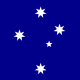# Add color terms for NB filters

XMLWordPrintable

## Details

• Type:Story
• Status: Done
• Resolution: Done
• Fix Version/s: None
• Component/s:
• Labels:
None
• Team:
Data Release Production

## Description

Furusawa-san writes:

I have determined the color terms for the new 3 narrow bands for PS1
bands. I feel like I would like to update only the 3 bands for now not
to destroy things in the other bands in this last minute change.
Do you agree with this idea? Or should we update all the other bands
as well for consistency?

 'N387': ColortermConfig.fromValues("g", "r", 0.549932112641, 1.93524092848, 0.335209939226), # fit to GS83 at g-r=(-1, 1.25) 'N718': ColortermConfig.fromValues("i", "r", -0.0374215790806, -0.293620763883, 0.190069559821), # fit to GS83 at g-r=(-1, 2) 'N973': ColortermConfig.fromValues("y", "z", -0.0064016547183, -0.0391528142634, -0.240885647814), # fit to GS83 at g-r=(-1.5, 0.5) 

altenatives

 'N718': ColortermConfig.fromValues("r", "i", -0.0374215792408, -0.706379236521, 0.190069560819), # fit to GS83 at g-r=(-1, 2) 'N973': ColortermConfig.fromValues("z", "i", 0.0126946008687, 0.282064376721, -0.0636499458066), # fit to GS83 at g-r=(-3, 3) 

## Activity

Thanks for agreeing to review this, Bob Armstrong. It's pretty simple:

 price@pap-laptop:~/LSST/obs_subaru (tickets/DM-10835=) $git sub-patch commit 0fdf0a33850641fea96739d46975a1375b1465da Author: Paul Price  Date: Tue Jun 6 15:28:28 2017 -0400    config: add hsc NB color terms    These values were calculated by Hisanori Furusawa from  fits to synthetic photometry from the Gunn-Stryker (1983)  atlas.    Fits were done over the following color ranges:  * N387: g-r=(-1, 1.25)  * N718: g-r=(-1, 2)  * N973: g-r=(-1.5, 0.5)   diff --git a/config/hsc/colorterms.py b/config/hsc/colorterms.py index 467c2ef..d64c4f4 100644 --- a/config/hsc/colorterms.py +++ b/config/hsc/colorterms.py @@ -29,7 +29,10 @@ config.data = {  'i2': Colorterm(primary="i", secondary="z", c0=0.00180361, c1=-0.18483562, c2=-0.02675511),  'z': Colorterm(primary="z", secondary="y", c0=-0.00907517, c1=-0.28840221, c2=-0.00316369),  'y': Colorterm(primary="y", secondary="z", c0=-0.00156858, c1=0.14747401, c2=0.02880125), + 'N387': Colorterm(primary="g", secondary="r", c0=0.54993211, c1=1.93524093, c2=0.33520994), + 'N718': Colorterm(primary="i", secondary="r", c0=-0.03742158, c1=-0.29362076, c2=0.19006956),  'N816': Colorterm(primary="i", secondary="z", c0=0.01191062, c1=-0.68757034, c2=-0.10781564),  'N921': Colorterm(primary="z", secondary="y", c0=0.00142051, c1=-0.59278367, c2=-0.25059679), + 'N973': Colorterm(primary="y", secondary="z", c0=-0.00640165, c1=-0.03915281, c2=-0.24088565),  }),  }  ShowPaul Price added a comment - Thanks for agreeing to review this, Bob Armstrong . It's pretty simple: price@pap-laptop:~/LSST/obs_subaru (tickets/DM-10835=)$ git sub-patch commit 0fdf0a33850641fea96739d46975a1375b1465da Author: Paul Price <price@astro.princeton.edu> Date: Tue Jun 6 15:28:28 2017 -0400   config: add hsc NB color terms These values were calculated by Hisanori Furusawa from fits to synthetic photometry from the Gunn-Stryker (1983) atlas. Fits were done over the following color ranges: * N387: g-r=(-1, 1.25) * N718: g-r=(-1, 2) * N973: g-r=(-1.5, 0.5)   diff --git a/config/hsc/colorterms.py b/config/hsc/colorterms.py index 467c2ef..d64c4f4 100644 --- a/config/hsc/colorterms.py +++ b/config/hsc/colorterms.py @@ -29,7 +29,10 @@ config.data = { 'i2': Colorterm(primary="i", secondary="z", c0=0.00180361, c1=-0.18483562, c2=-0.02675511), 'z': Colorterm(primary="z", secondary="y", c0=-0.00907517, c1=-0.28840221, c2=-0.00316369), 'y': Colorterm(primary="y", secondary="z", c0=-0.00156858, c1=0.14747401, c2=0.02880125), + 'N387': Colorterm(primary="g", secondary="r", c0=0.54993211, c1=1.93524093, c2=0.33520994), + 'N718': Colorterm(primary="i", secondary="r", c0=-0.03742158, c1=-0.29362076, c2=0.19006956), 'N816': Colorterm(primary="i", secondary="z", c0=0.01191062, c1=-0.68757034, c2=-0.10781564), 'N921': Colorterm(primary="z", secondary="y", c0=0.00142051, c1=-0.59278367, c2=-0.25059679), + 'N973': Colorterm(primary="y", secondary="z", c0=-0.00640165, c1=-0.03915281, c2=-0.24088565), }), }

## People

• Assignee:Paul Price
Reporter:Paul Price
Reviewers:
Bob Armstrong
Watchers:
Bob Armstrong, Paul Price